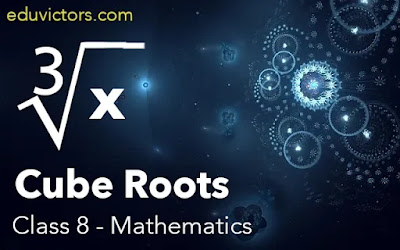# Class 8 - Maths - Cube Roots

Cube root is the inverse operation of a cube.

Symbol ∛ represents a cube-root.

e.g. $\sqrt{8} = 2$,

$\sqrt{216} = 6$

METHODS OF FINDING CUBE ROOT

There are two methods of finding Cube Root

1. Prime Factorisation

2. Estimation Method

1. Prime Factorisation method

A perfect cube can always be expressed as the product of triplets of equal prime factors.

To find cube root by prime factorisation, we need to perform the following steps:

Step 1: Express the given number into prime factors.

Step 2: Make groups in triplets of equal prime factors.

Step 3: Take one factor from each triplets of primes. Multiply them together.

Step 4: The product so obtained is the cube root of the given number.

Q1: Find cube root of 3375.

Answer: Prime factorisation of 3375 is:

3375 = 3 × 3 × 3 × 5 × 5 × 5

$\sqrt{3375} = \sqrt{{\color{Orchid}3\times3\times\3}{\color{Cyan}\times5\times5\times5}} = 3 \times 5 = 15$

Q2: Find cube root of 1728 by prime factorisation.

Answer: Prime factors of 1728 are:

$1728 = \underbrace{2 \times 2 \times 2}\times\underbrace{ 2 \times 2 \times 2} \times \underbrace{3 \times 3 \times 3}$

$\sqrt{1728} = 2\times 2 \times 3$

Q3: By what smallest number should 18252 be multiplied so that the product becomes a perfect cube? Find the cube root of the product.

Answer: Prime factors of 18252 is:

$18252 = 2 \times 2 \times \underbrace{3 \times 3 \times 3} \times 13 \times 13$

Here, 3 forms triplet, while 2 and 13 do not form triplet.

To make it a perfect cube, the number should be multiplied by  $2 \times 13=26$

$18252 = \underbrace{2 \times 2 \times 2} \times \underbrace{3 \times 3 \times 3} \times\underbrace{ 13 \times 13 \times 13}$

∴ $\sqrt{18252} = 2 \times 3 \times 13 = 78$

2. Estimation Method:

Follow the steps given below to understand this method:

Step 1: For given cube number start making groups of three digits starting from the right most digit of the number.

Step 2: First group will give you the one’s (or unit’s) digit of the required cube root.

Step3:Now take second group, let it be xyz. Find, a³ < xyz < b³.

We take the one’s place, of the smaller number a³ as the ten’s place of the required cube root.

Step 4: The digits obtained in step 2 and step 3 will give the final result.

Q4: Find the cube root of 17576 by Estimation Method

Step 1: Two groups are: $\overset{II}{17}-\overset{I}{576}$

Step 2: For the first group 576, the number at unit’s place will be 6.

Step 3: The second group is 17.

⇒ 8 < 17 < 27

⇒ 2³ <17<3³

We will take tens digit as 2

Step 4: Combining the two digits obtained in step 2 and 3, we get 26.

$\sqrt{17576} = 26$